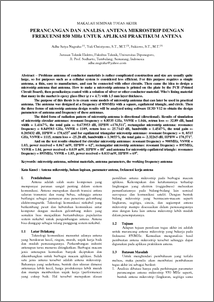# PERANCANGAN DAN ANALISA ANTENA MIKROSTRIP DENGAN FREKUENSI 850 MHz UNTUK APLIKASI PRAKTIKUM ANTENA

Nugraha, Adhe Setya and Christiyono, Yuli and Sukiswo, Sukiswo (2011) PERANCANGAN DAN ANALISA ANTENA MIKROSTRIP DENGAN FREKUENSI 850 MHz UNTUK APLIKASI PRAKTIKUM ANTENA. Undergraduate thesis, Diponegoro University.Preview
PDF - Published Version
739Kb

## Abstract

Problems antenna of conductor materials is rather complicated construction and size are usually quite large, so for purposes such as a cellular system is considered less efficient. For this purpose requires a simple antenna, a thin, easy to manufacture, and can be connected with other circuits. Then came the idea to design a microstrip antenna that antenna. How to make a microstrip antenna is printed on the plate by the PCB (Printed Circuit Board), then peradiasinya coated with a solution of silver or other conductor material. Who's lining material that many in the market is epoxy glass fiber ( r = 4.7) with 1.5 mm layer thickness. The purpose of this thesis is to create some models of microstrip antenna that can later be used in practical antenna. The antenna was designed at a frequency of 850MHz with a square, equilateral triangle, and circle. Then the three forms of microstrip antenna design results will be analyzed using software IE3D V12 to obtain the design parameters of antenna and frequency of these antennas. The third form of radiation pattern of microstrip antenna is directional (directional). Results of simulation of microstrip circular antennas: resonant frequency = 0.8535 GHz, VSWR = 1.046, retrun loss =- 32.89 dB, band width = 1.4167%, the total gain =- 0.673953 dB, HPBW =170,5110, rectangular microstrip antenna: resonance frequency = 0.849503 GHz, VSWR = 1109, return loss =- 25.7143 dB, bandwidth = 1.4547%, the total gain =- 0.205832 dB, HPBW = 170,6550 and for equilateral triangular microstrip antennas: resonant frequency = 0, 8515 GHz, VSWR = 1115, return loss = -25.28 dB, bandwidth = 1.3055%, the total gain =- 2.32265 dB, HPBW = 170,5710. And on the test results obtained for circular microstrip antennas: resonant frequency = 900MHz, VSWR = 1.03, power received = 0.067 mW, HPBW = 620, rectangular microstrip antennas: resonant frequency = 895MHz, VSWR = 1.04, power received = 0.039 mW, HPBW = 880 and antenna for microstrip equilateral triangle: resonance frequency = 895MHz, VSWR = 1.05, power received = 0.033 mW, HPBW = 690. Keywords: microstrip antenna, substrat materials, antenna parameters, the working frequency antenna Kata Kunci : Antena mikrostrip, bahan lapisan, paramater antena, frekuensi kerja antena

Item Type: Thesis (Undergraduate) T Technology > TK Electrical engineering. Electronics Nuclear engineering Faculty of Engineering > Department of Electrical EngineeringFaculty of Engineering > Department of Electrical Engineering 32063 Mr. Sudjadi Pranoto 20 Dec 2011 13:47 20 Dec 2011 13:47

Repository Staff Only: item control page